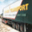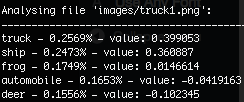# d246: TensorFlow CIFAR-10 tutorial, detailed step-by-step review, Part 2

### Execution process of ‘python cifar10_train.py’

1. Initialising flags and constants from cifar10.py and cifar10_input.py:
• data_dir
• batch_size
• use_fp16
• IMAGE_SIZE
• NUM_CLASSES
• NUM_EXAMPLES_PER_EPOCH_FOR_TRAIN
• NUM_EXAMPLES_PER_EPOCH_FOR_EVAL
• MOVING_AVERAGE_DECAY
• NUM_EPOCHS_PER_DECAY
• LEARNING_RATE_DECAY_FACTOR
• INITIAL_LEARNING_RATE
• Detailed description of all flags and constants: CIFAR-10 TensorFlow tutorial review, part 1
3. Calling the ‘cifar10_train.py‘ with default parameters.
1. Setting up the required variables.
2. Calling ‘distorted_inputs()‘ from ‘cifar10_input.py
• Filling the queue with 20’000 CIFAR images.
• Generating images and labels batch (shape: 128, 24, 24, 3; dtype:float32)
3. Calling ‘distorted_inputs()‘ from ‘cifar10.py to construct distorted input for CIFAR training.
4. Returns images to ‘cifar10_train.py/train()’
4. Building CIFAR-10 model ‘inference(images)‘ in ‘cifar10.py‘ with following layers:
• conv1
• pool1
• norm1
• conv2
• norm2
• pool2
• local3
• local4
• softmax
5. Defines ‘weight_decay‘ for each layer (tf.Variable).
6. Assigns the ‘loss_summaries‘ for each layer.
7. Builds and applies the gradients.
8. Builds histograms of weights and biases for each layer.
9. Creating a saver function, to be able to recover from checkpoints later.
10. Builds the summary operation.
11. Creates and starts the ‘tf.Session’.
12. Starting the queue runners, begins the training loop.

### Prediction (CIFAR-10 TensorFlow evaluation example outputs labels and probabilities)

Labels and probabilities output for single image of CIFAR-10 TensorFlow tutorial:

`from PIL import Image`

We will use the Python Imaging Library (PIL) to process images into JPEG files supported by out mode without additional losses.

```width = 24
height = 24

categories = [ "airplane","automobile","bird","cat","deer","dog","frog","horse","ship","truck" ]

filename = "/tmp/images/dog1.png" # absolute path to input image```
```im = Image.open(filename)
im.save(filename, format='JPEG', subsampling=0, quality=100)```

Converting the file from input format to JPEG with 100% quality and without subsampling.

```input_img = tf.image.decode_jpeg(tf.read_file(filename), channels=3)
tf_cast = tf.cast(input_img, tf.float32)```

Decoding image into 3 channels (red, green, blue) float32 array.

`float_image = tf.image.resize_image_with_crop_or_pad(tf_cast, height, width)`

Reshaping image.

`images = tf.expand_dims(float_image, 0)`

Creating a batch of images with just one image.

`logits = cifar10.inference(images)`

Get logits from main model (cifar10.py).

`_, top_k_pred = tf.nn.top_k(logits, k=5)`

Get top 5 predicions (‘top_k_pred’) and their values (‘_’).

`init_op = tf.initialize_all_variables()`

Initialising variables.

```with tf.Session() as sess:
sess.run(init_op)
_, top_indices = sess.run([_, top_k_pred])
for key, value in enumerate(top_indices):
print (categories[value] + ", " + str(_[key]))```

Runing tf.Session() and processing the output.

Example of input:Example of output: